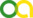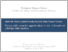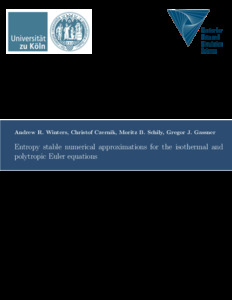Winters, Andrew R., Czernik, Christof, Schily, Moritz B. and Gassner, Gregor J. (2019). Entropy stable numerical approximations for the isothermal and polytropic Euler equations. Technical Report.Preview
PDF
CDS_TR-2019-15.pdf

## Abstract

In this work we analyze the entropic properties of the Euler equations when the system is closed with the assumption of a polytropic gas. In this case, the pressure solely depends upon the density of the fluid and the energy equation is not necessary anymore as the mass conservation and momentum conservation then form a closed system. Further, the total energy acts as a convex mathematical entropy function for the polytropic Euler equations. The polytropic equation of state gives the pressure as a scaled power law of the density in terms of the adiabatic index γ. As such, there are important limiting cases contained within the polytropic model like the isothermal Euler equations (γ = 1) and the shallow water equations (γ = 2). We first mimic the continuous entropy analysis on the discrete level in a finite volume context to get special numerical flux functions. Next, these numerical fluxes are incorporated into a particular discontinuous Galerkin (DG) spectral element framework where derivatives are approximated with summation-by-parts operators. This guarantees a high-order accurate DG numerical approximation to the polytropic Euler equations that is also consistent to its auxiliary total energy behavior. Numerical examples are provided to verify the theoretical derivations, i.e., the entropic properties of the high order DG scheme.

Item Type: Preprints, Working Papers or Reports (Technical Report)
Creators:
CreatorsEmailORCIDORCID Put Code
Winters, Andrew R.andrew.ross.winters@liu.seUNSPECIFIEDUNSPECIFIED
Czernik, Christofcczernik@math.uni-koeln.deUNSPECIFIEDUNSPECIFIED
Schily, Moritz B.mschily@math.uni-koeln.deUNSPECIFIEDUNSPECIFIED
Gassner, Gregor J.ggassner@math.uni-koeln.deUNSPECIFIEDUNSPECIFIED
URN: urn:nbn:de:hbz:38-97810
Series Name at the University of Cologne: Technical report series. Center for Data and Simulation Science
Volume: 2019,15
Date: 12 July 2019
Language: English
Faculty: Central Institutions / Interdisciplinary Research Centers
Divisions: Weitere Institute, Arbeits- und Forschungsgruppen > Center for Data and Simulation Science (CDS)
Subjects: Natural sciences and mathematics
Mathematics
Technology (Applied sciences)
Uncontrolled Keywords:
KeywordsLanguage
Isothermal EulerEnglish
Polytropic EulerEnglish
Entropy stabilityEnglish
Finite volumeEnglish
Summation-by-partsEnglish
Nodal discontinuous Galerkin spectral element methodEnglish
URI: http://kups.ub.uni-koeln.de/id/eprint/9781View Item